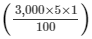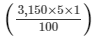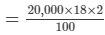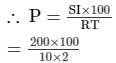# RD Sharma Solutions - Chapter 14 - Compound Interest (Part - 1), Class 8, Maths Notes | Study RD Sharma Solutions for Class 8 Mathematics - Class 8

## Class 8: RD Sharma Solutions - Chapter 14 - Compound Interest (Part - 1), Class 8, Maths Notes | Study RD Sharma Solutions for Class 8 Mathematics - Class 8

The document RD Sharma Solutions - Chapter 14 - Compound Interest (Part - 1), Class 8, Maths Notes | Study RD Sharma Solutions for Class 8 Mathematics - Class 8 is a part of the Class 8 Course RD Sharma Solutions for Class 8 Mathematics.
All you need of Class 8 at this link: Class 8

PAGE NO 14.4:

Question 1:

Find the compound interest when principal = Rs 3000, rate = 5% per annum and time = 2 years.

Principal for the first year = Rs 3,000

Interest for the first year = Rs= Rs 150

Amount at the end of the first year = Rs 3,000 + Rs 150

= Rs 3,150

Principal for the second year = Rs 3,150

Interest for the second year = Rs= Rs 157.50

Amount at the end of the second year = Rs 3,150 + Rs 157.50

= Rs 3307.50

∴ Compound interest = Rs(3,307.50 − 3,000)

= Rs 307.50

PAGE NO 14.4:

Question 2:

What will be the compound interest on Rs 4000 in two years when rate of interest is 5% per annum?

We know that amount A at the end of n years at the rate of R% per annum is given by A = P(1 + R/100)n.

Given:P = Rs 4,000

R = 5% p.a.

n = 2 years

Now,A = 4,000(1 + 5/100)²

= 4,000(1.05)²

= Rs 4,410

And,CI = A − P

= Rs 4,410 − Rs 4,000

= Rs 410

PAGE NO 14.4:

Question 3:

Rohit deposited Rs 8000 with a finance company for 3 years at an interest of 15% per annum. What is the compound interest that Rohit gets after 3 years?

We know that amount A at the end of n years at the rate of R% per annum is given by A = P(1 + R/100)n.

Given:P = Rs 8,000

R = 15% p.a.

n = 3 years

Now,A = 8,000(1 + 15/100)³

= 8,000(1.15)³

= Rs 12,167

And,CI = A − P

= Rs 12,167 − Rs 8,000

= Rs 4,167

PAGE NO 14.4:

Question 4:

Find the compound interest on Rs 1000 at the rate of 8% per annum for 1.5 years when interest is compounded half-yearly.

Given:P = Rs 1,000

R = 8% p.a.

n = 1.5 years

We know that:A = P(1 + R/200)²n

= 1,000(1 + 8/200)³

= 1,000(1.04)³

= Rs 1,124.86

Now,CI = A − P

= Rs 1,124.86 − Rs 1,000

= Rs 124.86

PAGE NO 14.4:

Question 5:

Find the compound interest on Rs 160000 for one year at the rate of 20% per annum, if the interest is compounded quarterly.

Given:P = Rs 16,000

R = 20% p.a.

n = 1 year

We know that:A = P(1 + R/400)4n

= 16,000(1 + 20/400)4

= 16,000(1.05)4

= Rs 19,448.1

Now,CI = A − P

= Rs 19,448.1 − Rs 16,000

= Rs 3,448.1

PAGE NO 14.5:

Question 6:

Swati took a loan of Rs 16000 against her insurance policy at the rate of 12.5% per annum. Calculate the total compound interest payable by Swati after 3 years.

Given:P = Rs 16,000

R = 12.5% p.a.

n = 3 years

We know that:A = P(1 + R/100)n

= 16,000(1 + 12.5/100)³

= 16,000(1.125)³ = Rs 22,781.25

Now, CI = A − P

= Rs 22,781.25 − Rs 16,000

= Rs 6,781.25

PAGE NO 14.5:

Question 7:

Roma borrowed Rs 64000 from a bank for 1.5 years at the rate of 10% per annum. Compute the total compound interest payable by Roma after 1.5 years, if the interest is compounded half-yearly.

Given:P = Rs 64,000

R = 10% p.a.

n = 1.5 years

Amount after n years:

A = P(1 + R/200)²

= 64,000(1 + 10/200)³

= 64,000(1.05)³

= Rs 74,088

Now,CI = A − P

= Rs 74,088 − Rs 64,000

= Rs 10,088

PAGE NO 14.5:

Question 8:

Mewa Lal borrowed Rs 20000 from his friend Rooplal at 18% per annum simple interest. He lent it to Rampal at the same rate but compounded annually. Find his gain after 2 years.

SI for Mewa Lal = PRT/100= Rs 7,200

Thus, he has to pay Rs 7,200 as interest after borrowing.

CI for Mewa Lal  =  A − P

= 20,000(1 + 18/100)² − 20,000

= 20,000(1.18)² − 20,000

= 27,848 − 20,000

= Rs 7,848

He gained Rs 7,848  as interest after lending.

His gain in the whole transaction = Rs 7,848 − Rs 7,200

= Rs 648

PAGE NO 14.5:

Question 9:

Find the compound interest on Rs 8000 for 9 months at 20% per annum compounded quarterly.

P = Rs 8,000

T = 9 months = 3 quarters

R = 20% per annum = 5% per quarter

A = 8,000(1 + 5/100)³

= 8,000(1.05)³

= 9,261

The required amount is Rs 9,261.

Now,CI = A − P

= Rs 9,261 − Rs 8,000

= Rs 1,261

PAGE NO 14.5:

Question 10:

Find the compound interest at the rate of 10% per annum for two years on that principal which in two years at the rate of 10% per annum gives Rs 200 as simple interest.

SI = PRT100Rs 1,000

A = P(1 + R/100)

= 1,000(1 + 10/100)²

= 1,000(1.10)² = Rs 1,210

Now,CI = A − P

= Rs 1,210 − Rs 1,000

= Rs 210

PAGE NO 14.5:

Question 11:

Find the compound interest on Rs 64000 for 1 year at the rate of 10% per annum compounded quarterly.

To calculate the interest compounded quarterly, we have:

A = P(1 + R/400)4n

= 64,000(1 + 10/400)4×1

= 64,000(1.025)

= 70,644.03

Thus, the required amount is Rs 70,644.03.

Now,CI = A − P

= Rs 70,644.025 − Rs 64,000

= Rs 6,644.03

PAGE NO 14.5:

Question 12:

Ramesh deposited Rs 7500 in a bank which pays him 12% interest per annum compounded quarterly. What is the amount which he receives after 9 months.

Given:P = Rs 7,500

R = 12% p.a. = 3% quarterly

T = 9 months = 3 quarters

We know that:A = P(1 + R/100)n

A = 7,500(1 + 3/100)³

= 7,500(1.03)³ = 8,195.45

Thus, the required amount is Rs 8,195.45.

PAGE NO 14.5:

Question 13:

Anil borrowed a sum of Rs 9600 to install a handpump in his dairy. If the rate of interest is 5.5% per annum compounded annually, determine the compound interest which Anil will have to pay after 3 years.

A = P(1 + R/100)

= 9,600(1 + 5.5/100)³

= 9,600(1.055)³ = Rs 11,272.72

Now,CI = A − P

= Rs 11,272.72 − Rs 9,600

= Rs 1,672.72

PAGE NO 14.5:

Question 14:

Surabhi borrowed a sum of Rs 12000 from a finance company to purchase a refrigerator. If the rate of interest is 5% per annum compounded annually, calculate the compound interest that Surabhi has to pay to the company after 3 years.

A = P(1 + R/100)

= 12,000(1 + 5/100)³

= 12,000(1.05)³ = 13,891.50

Thus, the required amount is Rs 13,891.50.

Now,CI = A − P

= Rs 13,891.50 − Rs 12,000

= Rs 1,891.50

PAGE NO 14.5:

Question 15:

Daljit received a sum of Rs. 40000 as a loan from a finance company. If the rate of interest is 7% per annum compounded annually, calculate the compound interest that Daljit pays after 2 years.

A = P(1 + R/100)n

= 40,000(1 + 7/100)²

= 40,000(1.07)²

= 45,796

Thus, the required amount is Rs 45,796.

Now,CI = A − P

= Rs 45,796 − Rs 40,000

= Rs 5,796

The document RD Sharma Solutions - Chapter 14 - Compound Interest (Part - 1), Class 8, Maths Notes | Study RD Sharma Solutions for Class 8 Mathematics - Class 8 is a part of the Class 8 Course RD Sharma Solutions for Class 8 Mathematics.
All you need of Class 8 at this link: Class 8Use Code STAYHOME200 and get INR 200 additional OFF

## RD Sharma Solutions for Class 8 Mathematics

88 docs

Track your progress, build streaks, highlight & save important lessons and more!

,

,

,

,

,

,

,

,

,

,

,

,

,

,

,

,

,

,

,

,

,

,

,

,

,

,

,

;